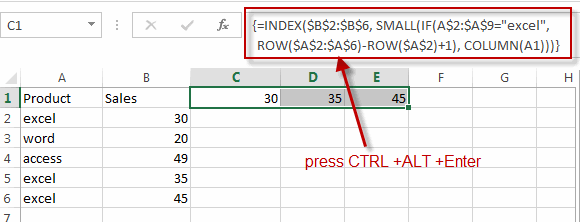# VLOOKUP Return Multiple Values Horizontally

This post will guide you how to vlookup a value and then return multiple corresponding values horizontally in Excel. How do I lookup a value and return multiple matched values in the same row in Excel. Or how to return multiple values with VLOOKUP function in Excel.

## VLOOKUP Return Multiple Values Horizontally

Assuming that you have a list of data that contain product name and product sales in the range A1:B6, and you want to vlookup a product “excel”” and return all sales value of the “excel” horizontally.

We have talked that the VLOOKUP function can be used to return only a corresponding value of the first occurrence of a lookup value. And if you want to return multiple values horizontally, how to achieve it.

You can create a complex array formula based on the INDEX function, the SMALL function, the IF function, the ROW function and the COLUMN function.

`=INDEX(\$B\$2:\$B\$6, SMALL(IF(A\$2:\$A\$9="excel", ROW(\$A\$2:\$A\$6)-ROW(\$A\$2)+1), COLUMN(A1)))`

Type this formula into a blank cell and then press Ctrl+Alt+Enter shortcuts to change this formula as array formula. And then drag the AutoFill handle from Cell C1 to E1.You will see that the multiple values have been extracted into the same row.

### Related Functions

• Excel SMALL function
The Excel SMALL function returns the smallest numeric value from the numbers that you provided. Or returns the smallest value in the array.The syntax of the SMALL function is as below:=SMALL(array,nth) …
• Excel INDEX function
The Excel INDEX function returns a value from a table based on the index (row number and column number)The INDEX function is a build-in function in Microsoft Excel and it is categorized as a Lookup and Reference Function.The syntax of the INDEX function is as below:= INDEX (array, row_num,[column_num])…
• Excel ROW function
The Excel ROW function returns the row number of a cell reference.The ROW function is a build-in function in Microsoft Excel and it is categorized as a Lookup and Reference Function.The syntax of the ROW function is as below:= ROW ([reference])….
• Excel IF function
The Excel IF function perform a logical test to return one value if the condition is TRUE and return another value if the condition is FALSE. The IF function is a build-in function in Microsoft Excel and it is categorized as a Logical Function.The syntax of the IF function is as below:= IF (condition, [true_value], [false_value])….
• Excel COLUMN function
The Excel COLUMN function returns the first column number of the given cell reference.The syntax of the COLUMN function is as below:=COLUMN ([reference])….
• Excel VLOOKUP function
The Excel VLOOKUP function lookup a value in the first column of the table and return the value in the same row based on index_num position.The syntax of the VLOOKUP function is as below:= VLOOKUP (lookup_value, table_array, column_index_num,[range_lookup])….

Related Posts

Build Hyperlink With VLOOKUP in Excel

You might have come across a task in which you were assigned to build hyperlinks, which seems very easy, and if you are new to excel or don't have enough experience with it, then you might wonder about doing this ...

Calculate Total Cost with Excel VLOOKUP Function

In today's article we will show you how to calculate the total cost for a given weight using the Excel VLOOKUP function. This function will help us to find the appropriate unit price for that weight and then we can ...

Basic Price Discount Calculation with Excel VLOOKUP Function

We often encounter product discounts in our shopping. Depending on the level of spending, the mall will offer different percentages of discounts. Usually, the more you spend, the bigger the discount, while the less you spend, the smaller the discount. ...

Calculate Grades With VLookup in Excel

Why Should You Calculate Grades With VLookup Excel? If you're looking for a simple way to find out a student's grades, VLOOKUP Excel can do the trick. This function uses a lookup table to find the values and sort them ...

Convert State Names To Abbreviations

Assume that you got a task to convert the full state's name into the abbreviations in MS Excel, and for doing this task, you might choose to do it manually, which is an acceptable way only if you don't have ...

Find and Replace Multiple Values

This post will guide you how to find and replace multiple values at once with VBA macro or using formula  in Excel. How do I make multiple find and replace in Excel. Suppose that you have a few cells containing ...

Excel XLOOKUP Function

Excel XLOOKUP Function was added into Excel as a beta feature in August 2019 and is now accessible exclusively in Microsoft 365. (as of July 2021). However, if you fall into this category and often deal with big sets of ...

VLOOKUP Formula | Faster Trick with 2 VLOOKUPS

This post will guide you how to use 2 VLOOKUPS function to looking up data entries from a given range of cells in Microsoft Excel. VLOOKUP with 2 lookups can be faster than a single VLOOKUP in certain scenarios. The ...

VBA Macro For VLOOKUP From Another Sheet

In the previous post, you should know that how to fix or remove the #N/A error when using VLOOKUP formula to lookup value from another sheet. And this post will show you how to use VBA code to vlookup data ...

VLOOKUP From Another Sheet Not Working

In the previous post, you should know that how to fix or remove the #N/A error when using VLOOKUP formula to lookup value from another sheet. And this post will show you reasons why your VLOOKUP formula is not working ...

Sidebar